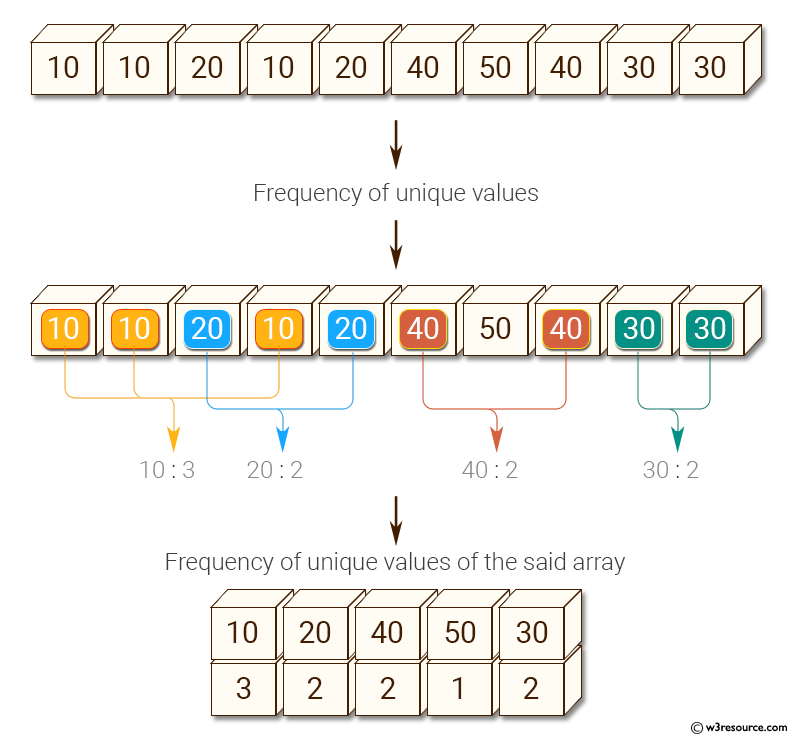﻿ NumPy: Count the frequency of unique values in numpy array - w3resource# NumPy: Count the frequency of unique values in numpy array

## NumPy: Array Object Exercise-94 with Solution

Write a NumPy program to count the frequency of unique values in numpy array.

Pictorial Presentation:Sample Solution:

Python Code:

``````import numpy as np
a = np.array( [10,10,20,10,20,20,20,30, 30,50,40,40] )
print("Original array:")
print(a)
unique_elements, counts_elements = np.unique(a, return_counts=True)
print("Frequency of unique values of the said array:")
print(np.asarray((unique_elements, counts_elements)))
```
```

Sample Output:

```Original array:
[10 10 20 10 20 20 20 30 30 50 40 40]
Frequency of unique values of the said array:
[[10 20 30 40 50]
[ 3  4  2  2  1]]
```

Python-Numpy Code Editor:

Have another way to solve this solution? Contribute your code (and comments) through Disqus.

What is the difficulty level of this exercise?

Test your Programming skills with w3resource's quiz.

﻿

## Python: Tips of the Day

Invokes the provided function after ms milliseconds:

Example:

```from time import sleep

def tips_delay(fn, ms, *args):
sleep(ms / 1000)
return fn(*args)
print(tips_delay(
lambda x: print(x),
1000,
'w3r'
))
```

Output:

```w3r
None
```